# VIT Entrance Exam Physics Sample Questions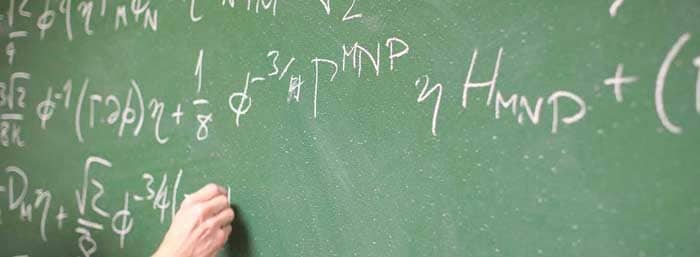40 multiple choice Physics questions for Vellore Institute of Technology Engineering Entrance Exam.

Ques: Carbon dating is best suited for determining the age of fossils if their age in years is of the order of
(a) 103
(b) 104
(c) 105
(d) 106

Ans. (b)

Ques. A transistor is used in common emitter mode as an amplifier. Then
(a) The base-emitter junction is forward biased
(b) The base-emitter junction is reverse biased
(c) The input signal is connected in series with the voltage applied to the base-emitter junction
(d) The input signal is connected in series with the voltage applied to bias the base collector junction

Ans. (a)
, (c)

Ques. When a magnet is brought near to a coil of metallic wire
(a) There is production of current which opposes the motion of the magnet
(b) There is production of current in the coil but it helps the motion of the magnet
(c) There is production of current in the coil but it neither helps nor opposes the motion of magnet
(d) There is no production of current in the coil

Ans. (a)

Ques. The Wrong statement about binding energy is
(a) It is the sum of the rest mass energies of nucleons minus the rest mass energy of the nucleus
(b) It is the energy released when the nucleons combine to form a nucleus
(c) It is the energy required to break a given nucleus into its constituent nucleus
(d) It is the sum of the kinetic energies of all the nucleons in the nucleus

Ans. (d)

Ques. The light rays having photons of energy 1.8 eV are falling on a metal surface having a work function 1.2 eV. What is the stopping potential to be applied to stop the emitting electrons
(a) 3 eV
(b) 1.2 eV
(c) 0.6 eV
(d) 1.4 eV

Ans. (c)

Ques. A ray of light incidents on a plane mirror at an angle of 30o. The deviation produced in the ray is
(a) 30o
(b) 60 o
(c) 90 o
(d) 120 o

Ans. (d)

Ques. The quantities conserved in nuclear reactions are
(a) Only charge
(b) Only linear momentum
(c) Only angular momentum
(d) All the above

Ans. (d)

Ques. There are two points A and B on the extended axis of a 2 cm bar magnet. Their distances from the centre of the magnet are x and 2x respectively. The ratio of magnetic fields at points A and B will be (approximately)
(a) 8:1
(b) 4:1
(c) 4:1
(d) 8:1

Ans. (a)

Ques. 56 tuning forks are placed in order of increasing frequency such that each tuning fork produces 4 beats per second with the nearest tuning fork. If the frequency of last tuning fork is three times that of the first tuning fork, then the frequency of first tuning fork will be
(a) 110 Hz
(b) 220 Hz
(c) 224 Hz
(d) 228 Hz

Ans. (a)

Ques. The fraction of a radioactive material which remains active after time is 9/16. The fraction which remains active after time t/2 will be
(a) 4/5
(b) 7/8
(c) 3/5
(d) ¾

Ans. (d)

Ques. A metal surface is illuminated by light of given intensity and frequency to cause photo-emission. If the intensity of illumination is reduced to one-fourth of its original value, then the maximum kinetic energy of emitted photo-electrons will become
(a) 1/16 of original value
(b) Unchanged
(c) Twice the original value
(d) Four times the original value

Ans. (b)

Related: Mechanical Waves MCQs

Ques. An X-ray tube operating at 50 kV converts 1% energy in the form of X-ray. If the amount of heat produced is 495 watt, then the number of electrons colliding with the target per second is
(a) 6.25 x 1016
(b) 4.15 x 1016
(c) 3.2 x 1016
(d) 1.2 x 1018

Ans. (a)

Ques. Water in an electric kettle boils off 15 minutes after the start of current flow. If the length of heating element is reduced to 2/3 rd of its initial length, then the time taken to boil off the same quantity of water at the same quantity of water at same electric voltage will be (in minutes)
(a) 10
(b) 15
(c) 30
(d) 60

Ans. (a)

Ques. Light waves can be polarised as they are
(a) Transverse
(b) Of high frequency
(c) Longitudinal
(d) Reflected

Ans. (a)

Ques. The aperture of a telescope is made large, because
(a) To increase the intensity of image
(b) To decrease the intensity of image
(c) To have greater magnification
(d) To have lesser resolution

Ans. (a)

Ques. A man standing in a swimming pool looks at a stone lying at the bottom. The depth of the swimming pool is h. At what distance from the surface of water is the image of the stone formed  (Line of vision is normal; Refractive index of water is n)
(a) h / n
(b) n / h
(c) h
(d) hn

Ans. (a)

Ques. For a given plate-voltage, the plate current in a triode is maximum when the potential of
(a) The grid is positive and plate is negative
(b) The grid is positive and plate is positive
(c) The grid is zero and plate is positive
(d) The grid is negative and plate is positive

Ans. (b)

Ques. In an X-rays tube, the intensity of the emitted X-rays beam is increased by
(a) Increasing the filament current
(b) Decreasing the filament current
(c) Increasing the target potential
(d) Decreasing the target potential

Ans. (a)

Ques. A ray of light is incidenting normally on a plane mirror. The angle of reflection will be
(a) 0 o
(b) 90 o
(c) Will not be reflected
(d) None of the above

Ans. (a)

Ques. The magnetic fields, through two identical rings made of copper and wood are changing at same rate. The induced electric field in copper ring will be
(a) More than that in the wooden ring
(b) Less than that in the wooden ring
(c) Finite and that in the wooden ring will be zero
(d) Same as that in the wooden ring

Ans. (d)

Ques. When a plane mirror is placed horizontally on a level ground at a distance of 60 m from the foot of a tower, the top of the tower and its image in the mirror subtend an angle of 90o at the eye. The height of the tower will be
(a) 30m
(b) 60m
(c) 90m
(d) 120m

Ans. (b)

Ques. Electromagnetic radiations described by the equation E = 100 [sin (2 x 1015) t + sin (6.28 x 1015)t] V/m are incident on a photosensitive surface having work function 2 eV. The maximum kinetic energy of photo-electrons will be
(a) 0.125 eV
(b) 2.125 eV
(c) 4.125 eV
(d) 6.125 eV

Ans. (b)

Ques. The radius of an air bubble becomes double when it rises from the bottom of a lake to its surface at constant temperature. If the atmospheric pressure is equal to that of water column of height, H, then the depth of the lake is
(a) 8 H
(b) 7 H
(c) H
(d) 2 H

Ans. (b)

Ques. Which of the following statement is wrong?
(a) Infrared photon has more energy than the photon of visible light
(b) Photographic plates are sensitive to ultraviolet rays
(c) Photographic plates can be made sensitive to infrared rays
(d) Infrared rays are invisible but can cast shadows like visible light rays

Ans. (a)

Ques. A block of mass 2 Kg. is lying on an inclined plane, inclined to the horizontal at an angle of 30°. The magnitude of frictional force acting on the block if the coefficient of friction between the block and the plane is 0.7, will be
(a) 11.9 Newton
(b) 1.19 Newton
(c) 0.119 Newton
(d) 1119 Newton

Ans. (a)

Ques. The temperature coefficient of resistance of a wire is 0.00125o C–1 At 300 K its resistance is one ohm. The resistance of the wire will be 2 ohm at
(a) 1154 K
(b) 1100 K
(c) 1400 K
(d) 1127 K

Ans. (d)

Ques. 10 ampere alternating current flows through the primary coil of transformer at 230 volt. If a voltage of 23000 volt is produced in the secondary coil and half of the power is lost in it, then the current in the secondary coil will be
(a) 0.05 A
(b) 0.5 A
(c) 0.1 A
(d) 1 A

Ans. (a)

Ques. The speed of a molecule of gas in a cubical vessel of side 5 meter is 15 ms/. This molecule is constantly colliding with the walls of the container. The collision frequency will be
(a) 0.2 per second
(b) 2.2.5 per second
(c) 5 per second
(d) 1.5 second

Ans. (d)

Ques. The mass of a proton is 1847 times that of an electron. An electron and a proton are injected into a uniform electric field at right angle to the direction of the filed with the same initial kinetic energy
(a) The electron trajectory will be less curved than the proton trajectory
(b) Both the trajectories will be straight
(c) The proton trajectory will be less curved than the electron trajectory
(d) Both the trajectories will be equally curved

Ans. (d)

Ques. A telescope with objective of focal length 60 cm and eye-piece of focal length 5 cm is focused on a far distance object such that parallel rays emerge from the eye-piece. If the object subtends an angle 2° on the objective, then angular width of image will be
(a) 10°
(b) 24°
(c) 50°
(d) 62°

Ans. (b)

Ques. A plane mirror makes an angle of 30o with horizontal. If a vertical ray strikes the mirror, find the angle between mirror and reflected ray
(a) 30 o
(b) 45 o
(c) 60 o
(d) 90 o

Ans. (c)

Related: Nuclear Chemistry Online Test

Ques. In Young’s double slit experiment, when a thin transparent sheet of thickness 2.5 x 10–3 cm is placed on one of the slits, then the interference pattern is displaced by a distance equal to 20 times of the fringe width. If the wave length of light used is 5000Å, then the refractive index of sheet is
(a) 2.3
(b) 1.95
(c) 1.6
(d) 1.4

Ans. (d)

Ques: A radioactive sample at any instant has its disintegration rate 5000 disintegration per minute. After 5 minutes, the rate is 1250 disintegrations per minute. Then, the decay constant (per minute) is
(a) 0.8 In 2
(b) 0.4 In 2
(c) 0.2 In 2
(d) 0.1 In 2

Ans. (b)

Ques. 8 cells are grouped to obtain the maximum current through a resistance of 2 ohm. If the emf of each cell is 2 volt and internal resistance is 1 ohm. Grouping of cells will have
(a) All cells in series
(b) All cells in parallel
(c) Two rows of four cells
(d) Four rows of two cells

Ans. (c)

Ques. A vessel contains a mixture of oxygen and hydrogen at room temperature. As compared to a hydrogen molecule, an oxygen molecule hits the wall
(a) With greater kinetic energy
(b) With smaller kinetic energy
(c) With greater average speed
(d) With smaller average speed

Ans. (d)

Ques. A photo cell is illuminated by a small bright source placed 1 m away. When the same source of light is placed 2 m away, the electrons emitted by photo cathode
(a) Each carry one quarter of the their previous energy
(b) Each carry one quarter of their previous momenta
(c) Are half numerous
(d) Are one-quarter as numerous

Ans. (d)

Ques. A surface ejects electrons when excited by green light but none when hited by yellow light. Will electrons be ejected if the surface is hited by red light
(a) Yes
(b) No
(c) Yes, if the red beam is quite intense
(d) Yes, if the red beam continues to fall upon the surface for a long time

Ans. (b)

Ques. In producing chlorine through electrolysis 100 KW power at 125 volt is being consumed. If the E.C.E. of chlorine is 0.367 x 10–6 kg/coul, then the mass of chlorine liberated per minute will be
(a) 16.3 x 10–4 kg
(b) 17.61 x 10–3 kg
(c) 18.2 x 10–3 kg
(d) 10–4 kg

Ans. (b)

Ques. For the four-bar linkage shown in the figure, the angular velocity of link AB is 1 rad/s. the length of link CD is 1.5 times the length of link AB. In the configuration shown, the angular velocity of link CD in rad/s is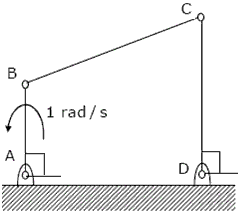(a) 3
(b) 3/2
(c) 1
(d) 2/3

Ans. (d)

Ques. Figure shows the schematic for the measurement of velocity of air (density = 1.2 kg/m) through a constant–area duct using a pitot tube and a water-tube manometer. The differential head of water (density = 1000 kg/m) in the two columns of the manometer is 10mm. Take acceleration due to gravity as 2 9.8m/ s . The velocity of air in m/s is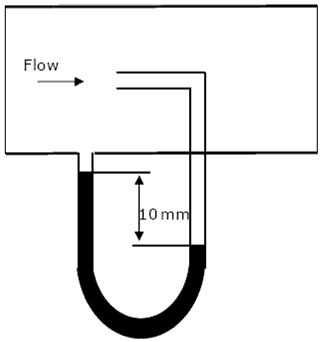(a) 6.4
(b) 9.0
(c) 12.8
(d) 25.6

Ans. (c)

Ques. A mass of 1kg is attached to two identical springs each with stiffness k = 20kN/m as shown in the figure. Under frictionless condition, the natural frequency of the system in Hz is close to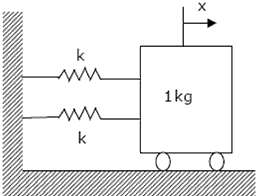(a) 32
(b) 23
(c) 16
(d) 11

Ans. (a)

Ques. A disc of mass m is attached to a spring of stiffness k as shown in the figure. The disc rolls without slipping on a horizontal surface. The natural frequency of vibration of the system is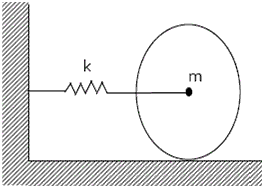(a)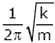(b)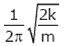(c)(d)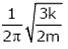Ans. (c)

Ques. A 1 kg block is resting on a surface with coefficient of friction µ = 0.1. A force of 0.8N is applied to the block as shown in figure. The friction force is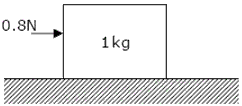(a) 0 N
(b) 0.8 N
(c) 0.98 N
(d) 1.2 N

Ans. (b)

Ques. A solid shaft of diameter, d and length L is fixed at both the ends. A torque, T0 is applied at a distance, L/4 from the left end as shown in the figure given below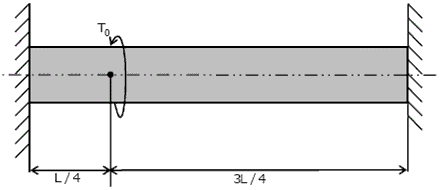The maximum shear stress in the shaft is

 (a)(b)(c)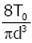(d)Ans. (b)

Ques. A uniform rigid rod of mass M and length L is hinged at one end as shown in the adjacent figure. A force P is applied at a distance of 2L/3 from the hinge so that the rod swings to the right. The reaction at the hinge is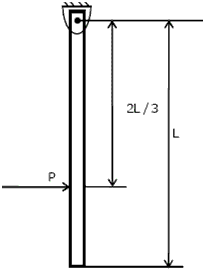(a) -P
(b) 0
(c) P/3
(d) 2P/3

Ans. (b)

### About the author

#### Richa Bhardwaj

Richa (B. Tech) has keen interest in Science and loves to teach students about it through lectures and assignments. She always try to use simple language and sentences while writing to make sure learner understands everything properly.